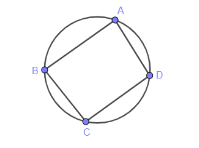QuestionAnswers

# Find the four angles of a cyclic quadrilateral ABCD in which $\angle A=\left( 2x-1 \right){}^\circ$ , $\angle B=\left( y+5 \right){}^\circ$ , $\angle C=\left( 2y+15 \right){}^\circ$ and $\angle D=\left( 4x-7 \right){}^\circ$ .(a) $\angle A=25{}^\circ ,\ \angle B=45{}^\circ ,\ \angle C=105{}^\circ ,\ \angle D=135{}^\circ$ (b) $\angle A=35{}^\circ ,\ \angle B=75{}^\circ ,\ \angle C=95{}^\circ ,\ \angle D=135{}^\circ$ (c) $\angle A=45{}^\circ ,\ \angle B=75{}^\circ ,\ \angle C=95{}^\circ ,\ \angle D=125{}^\circ$ (d) $\angle A=65{}^\circ ,\ \angle B=55{}^\circ ,\ \angle C=115{}^\circ ,\ \angle D=125{}^\circ$Verified
130.8k+ views
Hint: In a quadrilateral sum of opposite angles is always equal to ${{180}^{\circ }}$ , such as $\angle A+\angle C={{180}^{\circ }}$ and $\angle B+\angle D={{180}^{\circ }}$ . So, using this fact, we will equate all the expressions and from that we will find the value of x and y. Then by substituting the values of x and y in expressions of angles we will get our answer.

In question we are given equations of four angles of a quadrilateral and we are asked to find the value of angles. Now, we know that in a cyclic quadrilateral sum of opposite angles equal to ${{180}^{\circ }}$ . So, first of all we will draw a figure for our simplicity,Now, from figure we can see that $\angle A$ is an opposite angle to $\angle C$ and in the same way $\angle B$ is opposite angle to $\angle D$, so using the fact that sum of opposite angles in a quadrilateral is ${{180}^{\circ }}$ , we can see it mathematically as,
$\angle A+\angle C={{180}^{\circ }}$ ………….(i)
$\angle B+\angle D={{180}^{\circ }}$ ………….(ii)
Now, expressions of angles can be given as, $\angle A=\left( 2x-1 \right){}^\circ$ , $\angle B=\left( y+5 \right){}^\circ$ , $\angle C=\left( 2y+15 \right){}^\circ$ and $\angle D=\left( 4x-7 \right){}^\circ$ . So, on substituting these values in expression (i) and (ii), we will get,
$2x-1+2y+15={{180}^{\circ }}$ ………………(iii)
$y+5+4x-7={{180}^{\circ }}$ …………………(iv)
Now, we can make one of the two expressions similar to another expression, such as on multiplying expression (i) with 2 on both the sides we will get,
$2\left( 2x+2y+15-1 \right)=2\left( {{180}^{\circ }} \right)$
$4x+4y+28=360$
Now, making $4x$ as subject in above expression we will get,
$4x=360-28-4y\Rightarrow 4x=332-4y$ ………………(v)
Now, on substituting the value of $4x$ from expression (v) in expression (ii) we will get,
$y+5+332-4y-7={{180}^{\circ }}$
On, solving further we will get,
$330-3y=180\Rightarrow -3y=180-330$
$\Rightarrow -3y=-150$
$\Rightarrow y=\dfrac{-150}{-3}=50$ …………….(vi)
Now, on substituting value of y in expression (v) we will get,
$4x=332-4\left( 50 \right)$
$\Rightarrow x=\dfrac{332-200}{4}=\dfrac{132}{4}$
$\Rightarrow x=33$ …………..(vi)
Now, on substituting the values of X and Y in expressions of angles we will get,
$\angle A=\left( 2\left( 33 \right)-1 \right)=66-1={{65}^{\circ }}$
$\angle B=\left( 50+5 \right){}^\circ ={{55}^{\circ }}$
$\angle C=\left( 2\left( 50 \right)+15 \right){}^\circ =100+15={{115}^{\circ }}$
$\angle D=\left( 4\left( 33 \right)-7 \right){}^\circ =132-7={{125}^{\circ }}$
Hence, values of angles can be given as, $\angle A=65{}^\circ ,\ \angle B=55{}^\circ ,\ \angle C=115{}^\circ ,\ \angle D=125{}^\circ$ .
So, the correct answer is “Option D”.

Note: We know that the sum of opposite angles in quadrilateral is always equal to ${{180}^{\circ }}$, so we solved by equating the expression and then solving them. But instead of that we can also solve this sum by using option methods, such as, we know that $\angle A+\angle C={{180}^{\circ }}$ and $\angle B+\angle D={{180}^{\circ }}$ . So, we will consider the values given in option for example (a) and then we will substitute in expression as, $25+105=145\ne 180$ , in the same way we can find values of all options and at the end we will get option (d) as our correct answer because $65+115=180=180$. So, one can also solve by this method as it consumes less time and it is easy also.